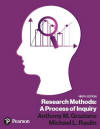﻿ Basic Two-Way ANOVAGraziano & Raulin
Research Methods (9th edition)

## Completely Randomized Factorial Designs

The beauty of ANOVA procedures is that they can be easily extended to more complex designs. This section will discuss a basic factorial design with two factors, both of which are between-subjects factors (See Chapter 12 of the textbook for details). The section closes with a brief introduction to even more complex designs, although covering such designs in detail is beyond the scope of this text.

### Simple Two-Way ANOVA

In a basic two-way ANOVA, there are two independent variables and they are crossed to form several independent groups. A two-way ANOVA is just the simplest of a set of ANOVA procedures for factorial designs, called factorial ANOVAs.

We will have to use slightly more complex notation here to describe the situation, but the notation is a simple extension of notation that we have used previously. We will call the two factors A and B (using capital letters), and we will use lowercase versions of those letters (a and b, respectively) to indicate the number of levels of each independent variable. Therefore, the total number of cells will be the number of levels of factor A times the number of levels of factor B.

When both factors are between-subjects factors (see Chapter 12 for details), we have three possible effects that we will evaluate in the ANOVA: (1) the main effect of factor A, (2) the main effect of factor B, and (3) the interaction of factors A and B.

The summary table below shows the formulas for everything except the sums of squares. The formulas for the sums of squares are shown in the unit that walks you through the manual computation of the two-way ANOVA. Click on the button below to view that unit.

It is technically possible to compute something as complex as a two-way ANOVA by hand. Thousands of graduate students from the 1940s through the 1970s routinely performed such computations on mechanical and crude electronic calculators. But with the ready availability of easy to use and inexpensive statistical analysis computer programs, such computations are quick and easy. If you want to see how to set up a two-way ANOVA using SPSS for Windows, click on the button below the summary table.

## ANOVA Summary Table for a Two-Way ANOVA

Source

df SS MS F p
Factor A dfA=a-1 SSA MSA=SSA/dfA

F=MSA/MSw

Factor B dfB=b-1 SSB MSB=SSB/dfB F=MSB/MSw
AB interaction dfAB=dfAXdfB SSAB MSAB=SSAB/dfAB F=MSAB/MSw
Within dfw=N-ab SSw MSw=SSw/dfw
Total dfT=N-1 SST

 Compute a Two-Way ANOVA  by hand Compute a Two-Way ANOVA using SPSS USE THE BROWSER'S BACK ARROW KEY TO RETURN

### More Complex ANOVAs

The real advantage of ANOVA is that it can be extended into almost any conceivable design, although the computation and the interpretation can become quite tedious as the complexity of the design increases.

For example, if we wanted to do a three-way ANOVA in which all of the factors were between-subjects factors, that is a simple extension of the two-way ANOVA above. We would have more sources of variance (A, B, C, AB, AC, BC, ABC, within, and Total). You could probably create a summary table for the three-way ANOVA like the one above showing the formulas for a two-way ANOVA by just looking at the pattern above and recreating it.

There is even an algorithm that you can use to create the long and complex formulas for the various sums of squares, which could be extended indefinitely to a five-way, or a ten-way, or even a 20-way ANOVAs, although in practice such complex ANOVAs would be unworkable and uninterruptible. For example, a ten-way ANOVA, assuming that there are only two levels of each factor, would require a minimum of 2048 participants (two each for each of 1024 cells). It would have 10 main effects, 45 two-way interactions, 120 three-way interactions, 210 four-way interactions, and so on.

The ANOVA can also be extended to situations that are called mixed designs (see Chapter 12 of the textbook). In statistically mixed designs, some of the factors are within-subjects factors and others are between-subjects factors. The SPSS for Windows program that can be bundled with this textbook (the student version) cannot handle the analysis of such designs, the but the full SPSS program can, and there are at least a dozen other statistical analysis programs on the market that can handle such analyses. It is beyond the scope of this text to show you how to set up such analyses, but it is useful for you to at least know the terminology that describes such designs and analyses.

The statistical analysis of mixed designs is typically described by stating how many of the factors are of each type. For example, a "two between, one within" analysis is used when two of the factors are between-subjects factors and one is a within-subjects factor. If all factors are within-subjects factors, the design is said to be a factorial repeated measures design. The formulas for such mixed designs are incredibly complex, but computers handle the analysis easily. However, the real problem is that few people alive have the capability of visualizing the kind of complex interactions that might be found in such analyses, which is the factor that provides the most severe limit on how far you can stretch factorial designs.